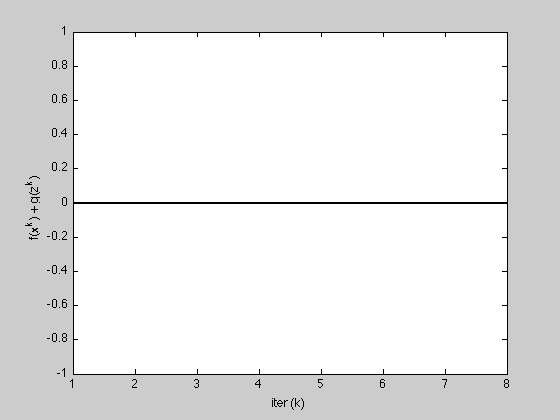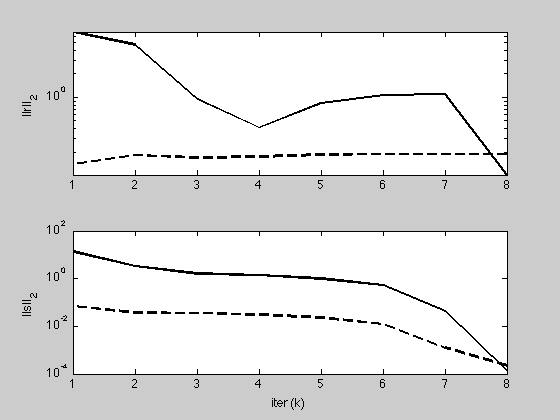## Contents

```% Find point in intersection of two polyhedra, given by
% { x | A1 x <= b1 } and { x | A2 x <= b2 }.
```

## Generate problem data

```randn('state', 0);
rand('state', 0);

n = 5;      % dimension of variable
m1 = 10;    % number of faces for polyhedra 1
m2 = 12;    % number of faces for polyhedra 2

c1 = 10*randn(n,1);        % center of polyhedra 1
c2 = -10*randn(n,1);       % center of polyhedra 2

% consider the following picture:
%
%       a1
% c ---------> x
%
% from the center "c", we travel along vector "a1" (not necessarily a unit
% vector) until we reach x. at "x", a1'x = b. a point y is to the left of x
% if a1'y <= b.
%

% pick m1 random directions with different magnitudes
A1 = diag(1 + rand(m1,1))*randn(m1,n);
% the value of b is found by traveling from the center along the normal
% vectors in A1 and taking its inner product with A1.
b1 = diag(A1*(c1*ones(1,m1) + A1'));

% pick m2 random directions with different magnitudes
A2 = diag(1 + rand(m2,1))*randn(m2,n);
% the value of b is found by traveling from the center along the normal
% vectors in A1 and taking its inner product with A1.
b2 = diag(A2*(c2*ones(1,m2) + A2'));

% find the distance between the two polyhedra--make sure they overlap by
% checking if the distance is 0
cvx_begin quiet
variables x(n) y(n)
minimize sum_square(x - y)
subject to
A1*x <= b1
A2*y <= b2
cvx_end

% if the distance is not 0, expand A1 and A2 by a little more than half the
% distance
if norm(x-y) > 1e-4,
A1 = (1 + 0.5*norm(x-y))*A1;
A2 = (1 + 0.5*norm(x-y))*A2;
% recompute b's as appropriate
b1 = diag(A1*(c1*ones(1,m1) + A1'));
b2 = diag(A2*(c2*ones(1,m2) + A2'));
end
```

## Solve problem

```[x history] = polyhedra_intersection(A1, b1, A2, b2, 1.0, 1.0);
```
```iter	    r norm	   eps pri	    s norm	  eps dual	 objective
1	    6.8713	    0.1385	   13.8243	    0.0689	      0.00
2	    4.6972	    0.1809	    3.3124	    0.0374	      0.00
3	    0.9614	    0.1692	    1.6155	    0.0354	      0.00
4	    0.4124	    0.1753	    1.3753	    0.0313	      0.00
5	    0.8436	    0.1839	    0.9909	    0.0229	      0.00
6	    1.0713	    0.1884	    0.5223	    0.0122	      0.00
7	    1.0909	    0.1888	    0.0446	    0.0012	      0.00
8	    0.1018	    0.1888	    0.0001	    0.0002	      0.00
Elapsed time is 2.392706 seconds.
```

## Reporting

```K = length(history.objval);

h = figure;
plot(1:K, history.objval, 'k', 'MarkerSize', 10, 'LineWidth', 2);
ylabel('f(x^k) + g(z^k)'); xlabel('iter (k)');

g = figure;
subplot(2,1,1);
semilogy(1:K, max(1e-8, history.r_norm), 'k', ...
1:K, history.eps_pri, 'k--',  'LineWidth', 2);
ylabel('||r||_2');

subplot(2,1,2);
semilogy(1:K, max(1e-8, history.s_norm), 'k', ...
1:K, history.eps_dual, 'k--', 'LineWidth', 2);
ylabel('||s||_2'); xlabel('iter (k)');
```## Compare to alternating projections

```% MAX_ITER = 10;
% x = zeros(n,1);
% z = zeros(n,1);
% for k = 1:MAX_ITER
%
%     % x-update
%     % use cvx to find point in first polyhedra
%     cvx_begin quiet
%         variable x(n)
%         minimize (sum_square(x - z))
%         subject to
%             A1*x <= b1
%     cvx_end
%
%     % z-update with relaxation
%     zold = z;
%     % use cvx to find point in second polyhedra
%     cvx_begin quiet
%         variable z(n)
%         minimize (sum_square(x - z))
%         subject to
%             A2*z <= b2
%     cvx_end
%
%     history1.r_norm(k)  = norm(x - z);
%     history1.s_norm(k)  = norm((z - zold));
%
% end
%
% g = figure
% subplot(2,1,1);
% semilogy(1:MAX_ITER, max(1e-8, history1.r_norm), 'k', 1:K, max(1e-8, history.r_norm), 'r');
% ylabel('||r||_2');
%
% subplot(2,1,2);
% semilogy(1:MAX_ITER, max(1e-8, history1.s_norm), 'k', 1:K, max(1e-8, history.s_norm), 'r');
% ylabel('||s||_2'); xlabel('iter (k)');
```